Next: Nearest-neighbor NMO Up: NORMAL MOVEOUT AND OTHER Previous: Looping over output space

## Formal inversion

We have thought of equation (10) as a formula for findingfrom.Now consider the opposite problem, findingfrom.Begin by multiplying equation (11) by the transpose matrix to define a new quantity:(12)
Obviously,is not the same as,but at least these two vectors have the same dimensionality. This turns out to be the first step in the process of findingfrom.Formally, the problem is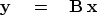(13)
And the formal solution to the problem is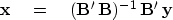(14)
Formally, we verify this solution by substituting (13) into (14).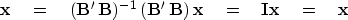(15)
In applications, the possible nonexistance of an inverse for the matrixis always a topic for discussion. For now we simply examine this matrix for the interpolation problem. We see that it is diagonal:(16)
So,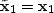; but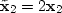.To recover the original data, we need to divide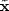by the diagonal matrix.Thus, matrix inversion is easy here.

Equation (14) has an illustrious reputation, which arises in the context of least squares.'' Least squares is a general method for solving sets of equations that have more equations than unknowns.

Recoveringfromusing equation (14) presumes the existence of the inverse of.As you might expect, this matrix is nonsingular when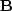stretches the data, because then a few data values are distributed among a greater number of locations. Where the transformation squeezes the data,must become singular, since returning uniquely to the uncompressed condition is impossible.

We can now understand why an adjoint operator is often an approximate inverse. This equivalency happens in proportion to the nearness of the matrixto an identity matrix. The interpolation example we have just examined is one in whichdiffers from an identity matrix merely by a scaling.Next: Nearest-neighbor NMO Up: NORMAL MOVEOUT AND OTHER Previous: Looping over output space
Stanford Exploration Project
10/21/1998